# Sample spaces and events

01/27/2021

## Instructions:

Use the left and right arrow keys to navigate the presentation forward and backward respectively. You can also use the arrows at the bottom right of the screen to navigate with a mouse.

FAIR USE ACT DISCLAIMER:
This site is for educational purposes only. This website may contain copyrighted material, the use of which has not been specifically authorized by the copyright holders. The material is made available on this website as a way to advance teaching, and copyright-protected materials are used to the extent necessary to make this class function in a distance learning environment. The Fair Use Copyright Disclaimer is under section 107 of the Copyright Act of 1976, allowance is made for “fair use” for purposes such as criticism, comment, news reporting, teaching, scholarship, education and research.

## Outline

• The following topics will be covered in this lecture:

• Random experiments
• Discrete and Continuous Sample Spaces
• Events and set notation
• Permutations and combinatinos

## Random experiments

• Suppose we conducting an experiment: measure the current in a thin copper wire.
• The measurements can differ slightly because of small variations in variables that are not controlled in our experiment, e.g.:
• changes in ambient temperatures;
• slight variations in the gauge;
• impurities in the chemical composition of the wire;
• current source drifts; and/or
• measurement errors.
• No matter how carefully an experiment is designed and conducted, the variation is almost always present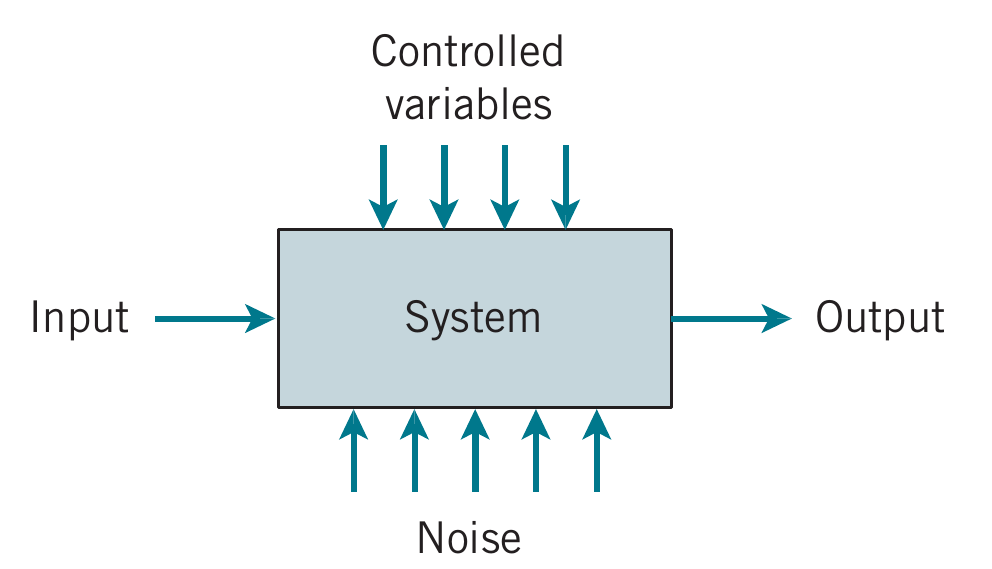Courtesy of Montgomery & Runger, Applied Statistics and Probability for Engineers, 7th edition

Our goal is to understand, quantify, and model the type of variations that we often encounter.

• Ours models incorporate uncontrollable inputs (noise) that combine with the controllable inputs to produce the output of our system.
• Because of the uncontrollable inputs, the same settings for the controllable inputs do not result in identical outputs every time the system is measured.

### Random experiments

Random experiment: an experiment that can result in different outcomes, even though it is repeated in the same manner every time.
• Example: in the design of a communication system, such as a voice communication network, the information capacity available to serve individuals using the network is an important design consideration.
• For voice communication, sufficient external lines need to be available to meet the requirements of a business.
• Assuming each line can carry only a single conversation, how many lines should be purchased?
• If too few lines are purchased, calls can be delayed or lost.
• The purchase of too many lines increases costs.
• Increasingly, design and product development is required to meet customer requirements at a competitive cost.
• In the design of the voice communication system, a model is needed for the number of calls and the duration of calls.
• Even knowing that, on average, calls occur every five minutes and that they last five minutes is not sufficient.
• If calls arrived precisely at five-minute intervals and lasted for precisely five minutes, one phone line would be sufficient.
• However, the slightest variation in call number or duration would result in some calls being blocked by others.
• A system designed without considering variation will be woefully inadequate for practical use.
• Our model for the number and duration of calls needs to include variation as an integral component.

### Sample spaces

The set of all possible outcomes of a random experiment is called the sample space of the experiment. The sample space is denoted as $$S$$.
• Consider an experiment that selects a cell phone camera and records the recycle time of a flash (the time taken to ready the camera for another flash).

• Because the time is positive, it is convenient to define the sample space as simply the positive real line $S=R^+ = \{x|\: x>0 \}$

• If it is known that all recycle times are between 1.5 and 5 seconds, the sample space can be $S = \{x|\: 1.5 < x < 5 \}$

• If the objective of the analysis is to consider only whether the recycle time is low, medium, or high $S=\{low,\:medium,\:high \}$

• If the objective is only to evaluate whether or not a particular camera conforms to a minimum recycle-time specification $S=\{yes,\:no \}$

• The sample space $$S$$ depends on the kinds of measurements we are taking as above.

### Discrete and Continuous Sample Spaces

• A sample space is continuous if it contains an interval (either finite or infinite) of real numbers.

• Recall the camera flash example, $$S = R^+$$ is an example of a continuous sample space.
• The unit of measurement can be arbitrarily sub-divided (seconds, miliseconds, etc.) and the measurement still makes sense.
• A sample space is discrete if it consists of a finite or countable infinite set of outcomes.

• Recall the camera flash example, $$S = \{yes,\: no\}$$ is a discrete sample space.
• The unit of measurement above cannot be arbitrarily sub-divided making the sample space discrete.
• The best choice of a sample space depends on the objectives of the study.

### Discrete and Continuous Sample Spaces

• Suppose that the recycle times of two cameras are recorded.

• The extension of the positive real line $$R$$ is to take the sample space to be the positive quadrant of the plane $S=R^+ \times R^+$

• Measurements would come in pairs of time units, one piece of data for each camera.

• If the objective of the analysis is to consider only whether or not the cameras conform to the manufacturing specifications, either camera may:

• (yes=y) conform; or
• may not conform (no=n)
• Then the sample space can be represented by the four outcomes: $S=\{yy,yn,ny,nn\}$

• If we are interested only in the number of conforming cameras in the sample, then the sample space can be $S=\{0,1,2\}$

### Tree diagrams

• Consider an experiment in which cameras are tested until the flash recycle time fails to meet the specifications. The sample space can be represented as $S=\{n, yn, yyn, yyyn, yyyyn, \text{and so forth}\}$
• Sample spaces can also be described graphically with tree diagrams when a sample space can be constructed in several steps or stages.
• Each of the $$n_1$$ ways of completing the first step as a branch of a tree.
• Each of the ways of completing the second step can be represented as $$n_2$$ branches starting from the ends of the original branches, and so forth.
• EXAMPLE: messages in a communication system are classified as to whether they are received within the time specified by the system design. The following a tree diagram represents the sample space of possible outcomes for three messages.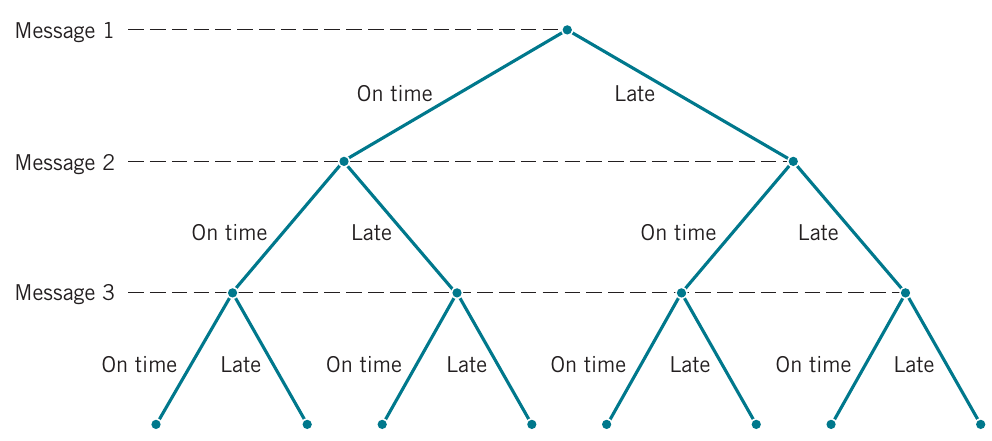Courtesy of Montgomery & Runger, Applied Statistics and Probability for Engineers, 7th edition

• Each message can be received either on time or late
• Stage 1: message 1
• $$n_1=2$$ ways of completing message
• Stage 2: message 2
• $$n_2=2$$ ways of completing message
• Stage 3: message 3
• $$n_3=2$$ ways of completing message

### Events

• An event is a subset of the sample space of a random experiment.
• Events are subsets, we can use basic set operations
• We can use Venn diagrams to represent a sample space and events in a sample space.
Union of two events is the event that consists of all outcomes that are contained in either of the two events.
Denoted $$A \cup B$$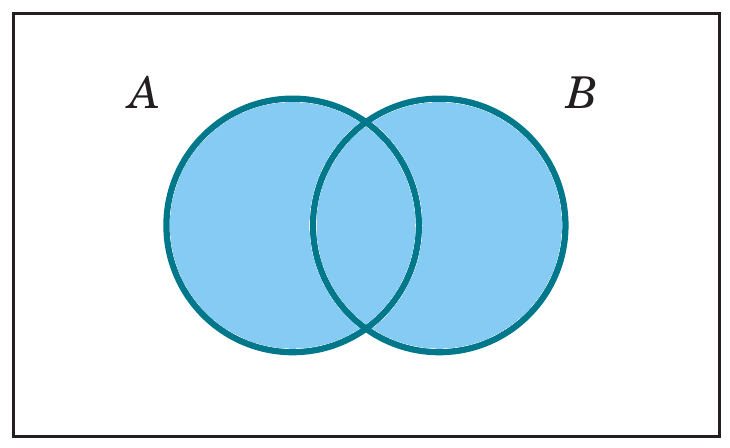Intersection of two events is the event that consists of all outcomes that are contained in both of the two events.
Denoted $$A \cap B$$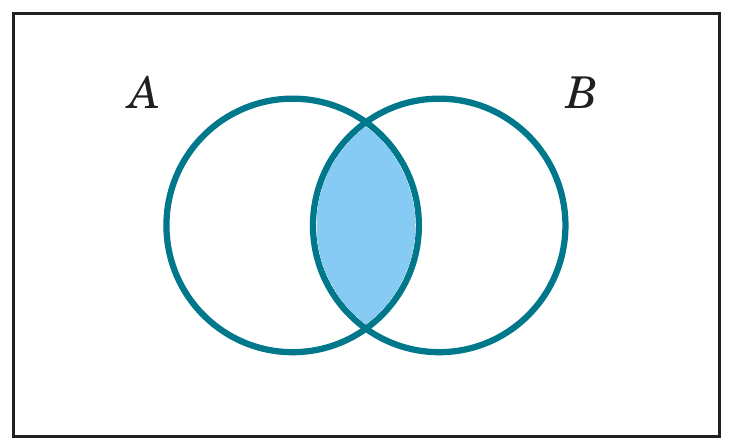Complement of an event in a sample space is the set of outcomes in the sample space that are not in the event.
Denoted $$A'$$ or $$A^c$$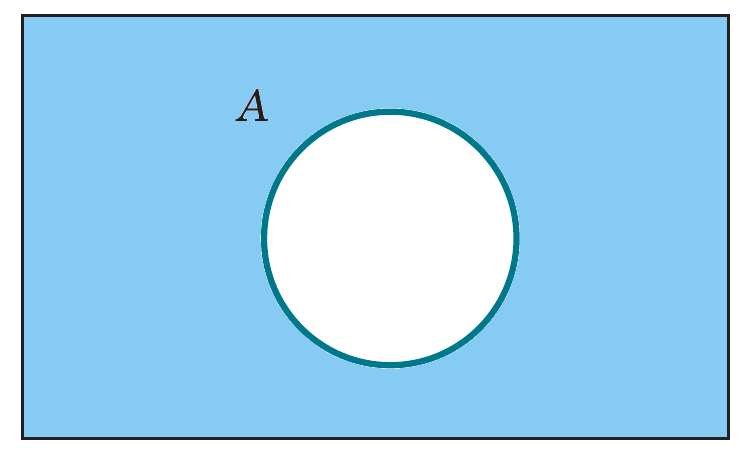### Camera flash events

• Recall the camera flash example with sample space $$S=\{yy,yn,ny,nn\}$$.
• Suppose that the subset of outcomes for which at least one camera conforms is denoted as $$E_1$$ $E_1 = \{yy, yn, ny\}$
• When the cameras do not conform, denote as event $$E_2$$, contains only the single outcome, $E_2 = \{nn\}$
• Other events are
• $$E_3 = \emptyset$$, the null set
• $$E_4 = S$$, the sample space
• Question: If $$E_5 = \{yn, ny, nn\}$$, what is …
• $$E_1 \cup E_5 =$$?
• $$E_1 \cup E_5 =S$$
• $$E_1 \cap E_5 =$$?
• $$E_1 \cap E_5 =\{yn,ny\}$$
• $$E_1' =$$?
• $$E_1' =\{nn\}$$